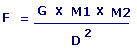Gravitational Force CalculatorG = Gravitational Constant M1 = Mass 1 M2 = Mass 2 D = Distance F = Gravitational Force

Click on Force in Dynes or Force in Newton you wish to calculate.

or

Enter value and click on calculate. Result will be displayed.

 Enter your values: : : :

 Results: :

 Web www.calculatoredge.com
 Theory of Universal gravitational is a physical law describing the gravitational attraction between massive bodies, described by Isaac Newton in 1687.

It states that: Every point mass attracts every other point mass by a force pointing along the line intersecting both points. The force is propotional to the product of the two masses and inversely propotional to the square of the distance between the point masses.

F is measured in Newtons, M1 and M2 in kilograms, D in metres and constant G is equal to 6.67 x 10-11 Nm2kg-2.

F can also be measured in Dynes, M1 and M2 in grams, D in centimetres and constant G is equal to 6.67 x 10E-8 Dyne.

Value of the constant G was first accurately determined from the results of Cavendish experiment conducted by british scientist Henry Cavendish in 1798.LINKSDISCLAIMERCONTACT US